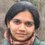# Gambler's ruin - 2

Continued from this note.

So far, we have examined the gambler's ruin problem on a small example. What can we say abut the more general case of starting out at some budget $k$ and deciding to cash out at some threshold $n$?

You walk into Martin Gale's Betting Room with an initial budget of $k$

As usual, you can play the following game any number of times (if you have what it costs)

• You pay Martin a dollar.
• Martin tosses a fair coin
• If the coin comes up heads Martin pays you two dollars. If it comes up tails, you get nothing.

You decide that you will play until you have increased your money to $n$, and then you will stop. Here, $n>k$. Of course you will also have to stop if you lose all your money (i.e. you are ruined).

What is the probability that you are ruined?

There are the number of ways to tackle this, but the approach I'm going to take is to set up a system of linear equations. Let $r_{k,n}$ denote the probability we want to compute, namely the probability that, starting out at a budget of $k$ you will lose all your money before you ever hit $n$.

Since it is pessimistic to talk about ruin, let's set up the system in terms of variables representing the probabilities of winning, i.e. the probability of cashing out at $n$. In what follows, we will keep $n$ fixed, and so we will subscript the variables with only the budget.

For $0 \le i \le n$, let $w_{i}$ be the probability that you cash out, starting from an initial budget of $i$. We want to compute $w_{k}$. If you play the game once, then with probability 1/2 you increase your budget by 1 and with probability 1/2 you decrease it by one. And then you get to play again, as if you were starting with your new budget. Thus

• $w_0 =0$ -- If you have no money you can't play, and therefore can't win.
• $w_{i} = \frac{1}{2} (w_{i-1} + w_{i+1})$ for $1\le i\le n-1$
• $w_{n} = 1$ -- Because of your decision to take the money and quit when you reach $n$.

This gives a system of linear equations in $w_0 \dots w_n$. Combining the first two of these we easily see that $w_2 = 2w_1$ We will use this as a base case of an induction. Now suppose it is true that $w_{i} = i w_1$ for all $i. Then we'll show it is also true for $j$ as follows: We know that $w_{j-1} = \frac{w_{j-2}+w_{j}}{2} \,.$ Using the induction hypothesis to substitute for $w_{j-1}$ and $w_{j-2}$ we have $w_{j} = 2(j-1)w_1 - (j-2) w_1 = j w_1 \,.$ But now, using the fact that $w_n =1$, we have $w_1 = \frac{1}{n}$, and finally $w_k = \frac{k}{n}$ It follows that the probability of ruin is $\frac{n-k}{n}$.

Now what about the question of how long you play for?

You walk into Martin Gale's Betting Room with an initial budget of $k$

As usual, you can play the following game any number of times (if you have what it costs)

• You pay Martin a dollar.
• Martin tosses a fair coin
• If the coin comes up heads Martin pays you two dollars. If it comes up tails, you get nothing.

You decide that you will play until you have increased your money to $n$, and then you will stop. Here, $n>k$. Of course you will also have to stop if you lose all your money (i.e. you are ruined).

How many games do you expect to play before you stop?

Again, setting up a system of linear equations solves the problem. This time the equations we have are - $g_0 = g_n =0$ and - $g_{i} = 1+ \frac{ g_{i-1} + w_{g+1}}{2}$ for $1\le i\le n-1$ where $g_i$ is the expected number of games starting at a budget of $i$. It is not too hard to solve this and see that we get $g_k = k(n-k)$

To be continued...Note by Varsha Dani
2 years, 5 months ago

This discussion board is a place to discuss our Daily Challenges and the math and science related to those challenges. Explanations are more than just a solution — they should explain the steps and thinking strategies that you used to obtain the solution. Comments should further the discussion of math and science.

When posting on Brilliant:

• Use the emojis to react to an explanation, whether you're congratulating a job well done , or just really confused .
• Ask specific questions about the challenge or the steps in somebody's explanation. Well-posed questions can add a lot to the discussion, but posting "I don't understand!" doesn't help anyone.
• Try to contribute something new to the discussion, whether it is an extension, generalization or other idea related to the challenge.

MarkdownAppears as
*italics* or _italics_ italics
**bold** or __bold__ bold
- bulleted- list
• bulleted
• list
1. numbered2. list
1. numbered
2. list
Note: you must add a full line of space before and after lists for them to show up correctly
paragraph 1paragraph 2

paragraph 1

paragraph 2

[example link](https://brilliant.org)example link
> This is a quote
This is a quote
    # I indented these lines
# 4 spaces, and now they show
# up as a code block.

print "hello world"
# I indented these lines
# 4 spaces, and now they show
# up as a code block.

print "hello world"
MathAppears as
Remember to wrap math in $$ ... $$ or $ ... $ to ensure proper formatting.
2 \times 3 $2 \times 3$
2^{34} $2^{34}$
a_{i-1} $a_{i-1}$
\frac{2}{3} $\frac{2}{3}$
\sqrt{2} $\sqrt{2}$
\sum_{i=1}^3 $\sum_{i=1}^3$
\sin \theta $\sin \theta$
\boxed{123} $\boxed{123}$